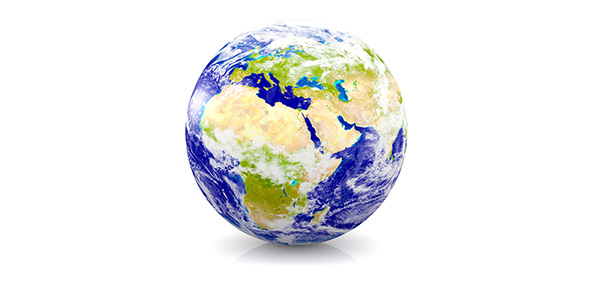# Do You Really Know About Earth's Physical Structure? Trivia Quiz

12 Questions | Total Attempts: 32SettingsThis is quiz has been developed for students and learners to gain knowledge about earthquakes, plate tectonics, and their effects. Do attempt all the questions So, let's try out the quiz. All the best!

Related Topics
• 1.
Stress is a____.
• A.

Force in a push or a pull

• 2.
What are body waves?
• A.

Surface waves

• B.

P-waves

• C.

S-waves

• D.

B and C

• E.

All of the above

• 3.
What is a fault?
• A.

A crack in a tree

• B.

A crack in rocks where rocks break

• C.

Where lava comes out

• D.

None of the above

• 4.
What is compression?
• A.

Pulling apart

• B.

Squeezing together

• C.

A twisting motion

• D.

Sliding past somethin

• 5.
What is the epicentre?
• A.

None of the above

• B.

The place a quake actuly accurs

• C.

A place where there is an I.E.P.

• D.

The place where the quake seems to come from

• 6.
Name the terms for small and large depressions.
• A.

Craters and faults

• B.

Trenches and caldaras

• C.

Craters and caldaras

• D.

Faults and trenches

• E.

None of the above

• 7.
What is the name for molten rock?
• A.

Lava

• B.

Fluid

• C.

Mantle

• D.

None of the above

• 8.
What are the name for the crust and upper mantle?
• A.

Lithosphere

• B.

Genosphere

• C.

Asthenosphere

• D.

Sciosphere

• 9.
___ is a crack in the earth's crust?
• A.

Vent

• 10.
___ is also known as the "flying" rock.
• A.

Tefera

• 11.
The places where plates meet is called what?
• A.

Bounderies

• B.

Rings

• C.

Belts

• D.

Trenches

• 12.
True or false, A (cinder-cone) is a mountain with shallow sides.
• A.

True

• B.

False